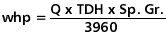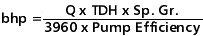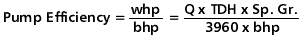### PowerandEfficiency

The work performed by a pump is a function of the total head and the weight of the liquid pumped in a given time period. The pump capacity in gpm and the liquid specific gravity are normally used in the formulas rather than the actual weight of the liquid pumped.

Pump input or brake horsepower (bhp) is the actual horsepower delivered to the pump shaft. Pump output or hydraulic horsepower (whp) is the liquid horsepower delivered by the pump. These two terms are defined by the following formulas.The constant 3960 is obtained by dividing the number or foot pounds for one horsepower (33,000) by the weight of one gallon of water (8.33 pounds.)

The brake horsepower or input to a pump is greater than the hydraulic horsepower or output due to the mechanical and hydraulic losses incurred in the pump. Therefore the pump efficiency is the ratio of these two values.